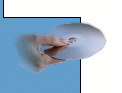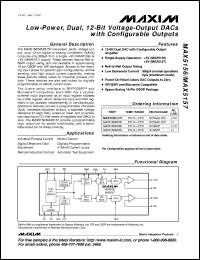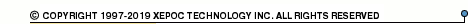More than478 253 registered clientsMAX5171 series datasheets. Manufacturer: Maxim Integrated Products.

 MAX5171AEEE Low-power, serial, 14-bit DAC with force/sence voltage output. Single-supply +5V operation. Full-scale output range +2.048V(Vref=+1.25V). INL(LSB) +-1. in 16-pin QSOP package. Operational temperature range from -40°C to 85°C. Datasheet*) MAX5171BEEE Low-power, serial, 14-bit DAC with force/sence voltage output. Single-supply +5V operation. Full-scale output range +2.048V(Vref=+1.25V). INL(LSB) +-2. in 16-pin QSOP package. Operational temperature range from -40°C to 85°C. Datasheet*) MAX5173AEEE Low-power, serial, 14-bit DAC with force/sence voltage output. Single-supply +3V operation. Full-scale output range +4.096V(Vref=+2.5V). INL(LSB) +-2. in 16-pin QSOP package. Operational temperature range from -40°C to 85°C. Datasheet*) MAX5173BEEE Low-power, serial, 14-bit DAC with force/sence voltage output. Single-supply +3V operation. Full-scale output range +4.096V(Vref=+2.5V). INL(LSB) +-4. in 16-pin QSOP package. Operational temperature range from -40°C to 85°C. Datasheet*) MAX5171 Low-Power, Serial, 14-Bit DACs with Force/Sense Voltage Output Datasheet*) MAX5171AEEE+ Low-Power, Serial, 14-Bit DACs with Force-Sense Voltage-Output. Lead-free Datasheet*) MAX5171AEEE+T Low-Power, Serial, 14-Bit DACs with Force-Sense Voltage-Output. Lead-free Datasheet*) MAX5171AEEE-T Low-Power, Serial, 14-Bit DACs with Force-Sense Voltage-Output Datasheet*) MAX5171BEEE+ Low-Power, Serial, 14-Bit DACs with Force-Sense Voltage-Output. Lead-free Datasheet*) MAX5171BEEE+T Low-Power, Serial, 14-Bit DACs with Force-Sense Voltage-Output. Lead-free Datasheet*) MAX5171BEEE-T Low-Power, Serial, 14-Bit DACs with Force-Sense Voltage-Output Datasheet*) MAX5173AEEE+ Low-Power, Serial, 14-Bit DACs with Force-Sense Voltage-Output. Lead-free Datasheet*) MAX5173AEEE+T Low-Power, Serial, 14-Bit DACs with Force-Sense Voltage-Output. Lead-free Datasheet*) MAX5173AEEE-T Low-Power, Serial, 14-Bit DACs with Force-Sense Voltage-Output Datasheet*) MAX5173BEEE+ Low-Power, Serial, 14-Bit DACs with Force-Sense Voltage-Output. Lead-free Datasheet*) MAX5173BEEE+T Low-Power, Serial, 14-Bit DACs with Force-Sense Voltage-Output. Lead-free Datasheet*) MAX5173BEEE-T Low-Power, Serial, 14-Bit DACs with Force-Sense Voltage-Output Datasheet*)